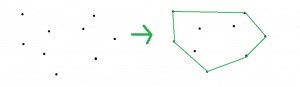# Convex Hull | Monotone chain algorithm

Given a set of points, the task is to find the covex hull of the given points. The convex hull is the smallest convex polygon that contains all the points.Examples:

Input: Points[] = {{0, 3}, {2, 2}, {1, 1}, {2, 1}, {3, 0}, {0, 0}, {3, 3}}
Output:
(0, 0)
(3, 0)
(3, 3)
(0, 3)

## Recommended: Please try your approach on {IDE} first, before moving on to the solution.

Approach: Monotone chain algorithm constructs the convex hull in O(n * log(n)) time. We have to sort the points first and then calculate the upper and lower hulls in O(n) time. The points will be sorted with respect to x-coordinates (with respect to y-coordinates in case of a tie in x-coordinates), we will then find the left most point and then try to rotate in clockwise direction and find the next point and then repeat the step until we reach the rightmost point and then again rotate in the the clockwise direction and find the lower hull.

Below is the implementation of the above approach:

 `// C++ implementation of the approach ` `#include ` `#define llu long long int ` `using` `namespace` `std; ` ` `  `struct` `Point { ` ` `  `    ``llu x, y; ` ` `  `    ``bool` `operator<(Point p) ` `    ``{ ` `        ``return` `x < p.x || (x == p.x && y < p.y); ` `    ``} ` `}; ` ` `  `// Cross product of two vectors OA and OB ` `// returns positive for counter clockwise ` `// turn and negative for clockwise turn ` `llu cross_product(Point O, Point A, Point B) ` `{ ` `    ``return` `(A.x - O.x) * (B.y - O.y) ` `           ``- (A.y - O.y) * (B.x - O.x); ` `} ` ` `  `// Returns a list of points on the convex hull ` `// in counter-clockwise order ` `vector convex_hull(vector A) ` `{ ` `    ``int` `n = A.size(), k = 0; ` ` `  `    ``if` `(n <= 3) ` `        ``return` `A; ` ` `  `    ``vector ans(2 * n); ` ` `  `    ``// Sort points lexicographically ` `    ``sort(A.begin(), A.end()); ` ` `  `    ``// Build lower hull ` `    ``for` `(``int` `i = 0; i < n; ++i) { ` ` `  `        ``// If the point at K-1 position is not a part ` `        ``// of hull as vector from ans[k-2] to ans[k-1]  ` `        ``// and ans[k-2] to A[i] has a clockwise turn ` `        ``while` `(k >= 2 && cross_product(ans[k - 2],  ` `                          ``ans[k - 1], A[i]) <= 0) ` `            ``k--; ` `        ``ans[k++] = A[i]; ` `    ``} ` ` `  `    ``// Build upper hull ` `    ``for` `(``size_t` `i = n - 1, t = k + 1; i > 0; --i) { ` ` `  `        ``// If the point at K-1 position is not a part ` `        ``// of hull as vector from ans[k-2] to ans[k-1]  ` `        ``// and ans[k-2] to A[i] has a clockwise turn ` `        ``while` `(k >= t && cross_product(ans[k - 2], ` `                           ``ans[k - 1], A[i - 1]) <= 0) ` `            ``k--; ` `        ``ans[k++] = A[i - 1]; ` `    ``} ` ` `  `    ``// Resize the array to desired size ` `    ``ans.resize(k - 1); ` ` `  `    ``return` `ans; ` `} ` ` `  `// Driver code ` `int` `main() ` `{ ` `    ``vector points; ` ` `  `    ``// Add points ` `    ``points.push_back({ 0, 3 }); ` `    ``points.push_back({ 2, 2 }); ` `    ``points.push_back({ 1, 1 }); ` `    ``points.push_back({ 2, 1 }); ` `    ``points.push_back({ 3, 0 }); ` `    ``points.push_back({ 0, 0 }); ` `    ``points.push_back({ 3, 3 }); ` ` `  `    ``// Find the convex hull ` `    ``vector ans = convex_hull(points); ` ` `  `    ``// Print the convex hull ` `    ``for` `(``int` `i = 0; i < ans.size(); i++) ` `        ``cout << ``"("` `<< ans[i].x << ``", "`  `             ``<< ans[i].y << ``")"` `<< endl; ` ` `  `    ``return` `0; ` `} `

Output:

```(0, 0)
(3, 0)
(3, 3)
(0, 3)
```My Personal Notes arrow_drop_upCheck out this Author's contributed articles.

If you like GeeksforGeeks and would like to contribute, you can also write an article using contribute.geeksforgeeks.org or mail your article to contribute@geeksforgeeks.org. See your article appearing on the GeeksforGeeks main page and help other Geeks.

Please Improve this article if you find anything incorrect by clicking on the "Improve Article" button below.